# Qubit, An Intuition #1 — First Baby Steps in Exploring the Quantum World

`TL;DR; - The intuition of a quantum bit (qubit) as a computing unit in a Quantum computer, in comparison to a binary digit (bit) in a Classical computer.- Bra-ket notation, bloch sphere, hybrid Classical-Quantum.- The source code (in Python) for generating illustrated qubit state transitions is available on github.- This is the first article in the "Qubit, An Intuition" series. Upcoming articles (towards the end of 2021) will discuss "Inner Product, Outer Product, and Tensor Product", "Quantum Measurement", "Unitary Matrices", "Quantum Circuit and Reversible Transformation", as well as "Quantum Algorithms."`

For an introductory helicopter view of the overall six articles in the series, please visit this link “Embarking on a Journey to Quantum Computing — Without Physics Degree.”IBM Quantum computers (IBM, 2021d)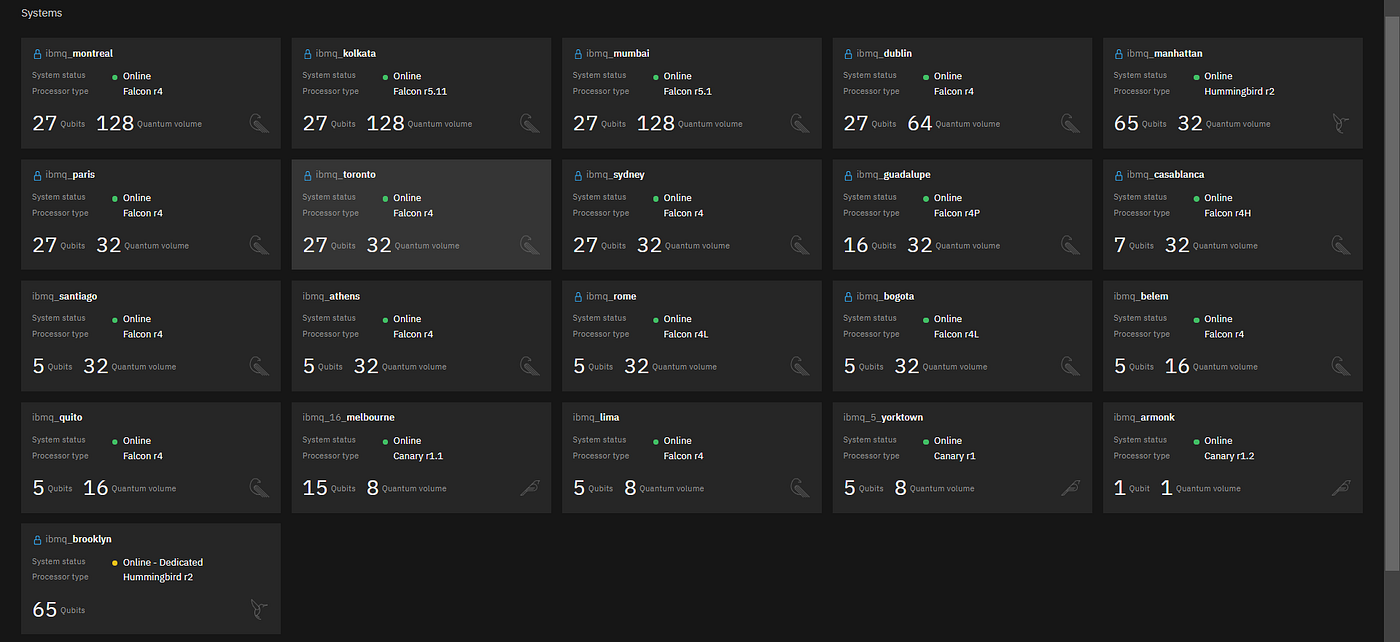The operating status of 26 IBM real Quantum computers. The quantum computers are accessible from the IBM Cloud (as of May 12, 2021).

# Quantum bit (Qubit)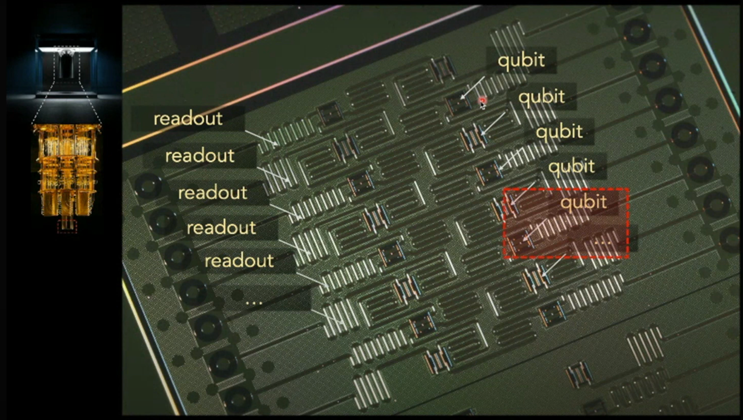A physical qubit realization in IBM Quantum chip, based on superconducting qubit (Zlatko Minev, 2020).
`“The average T1 time of a qubit is the time it takes for a qubit to decay from the excited state to the ground state. It is important because it limits the duration of meaningful programs we can run on the quantum computer.,” stated in Qiskit Textbook (IBM, 2021b) within the section titled “Calibrating Qubits with Qiskit Pulse.”`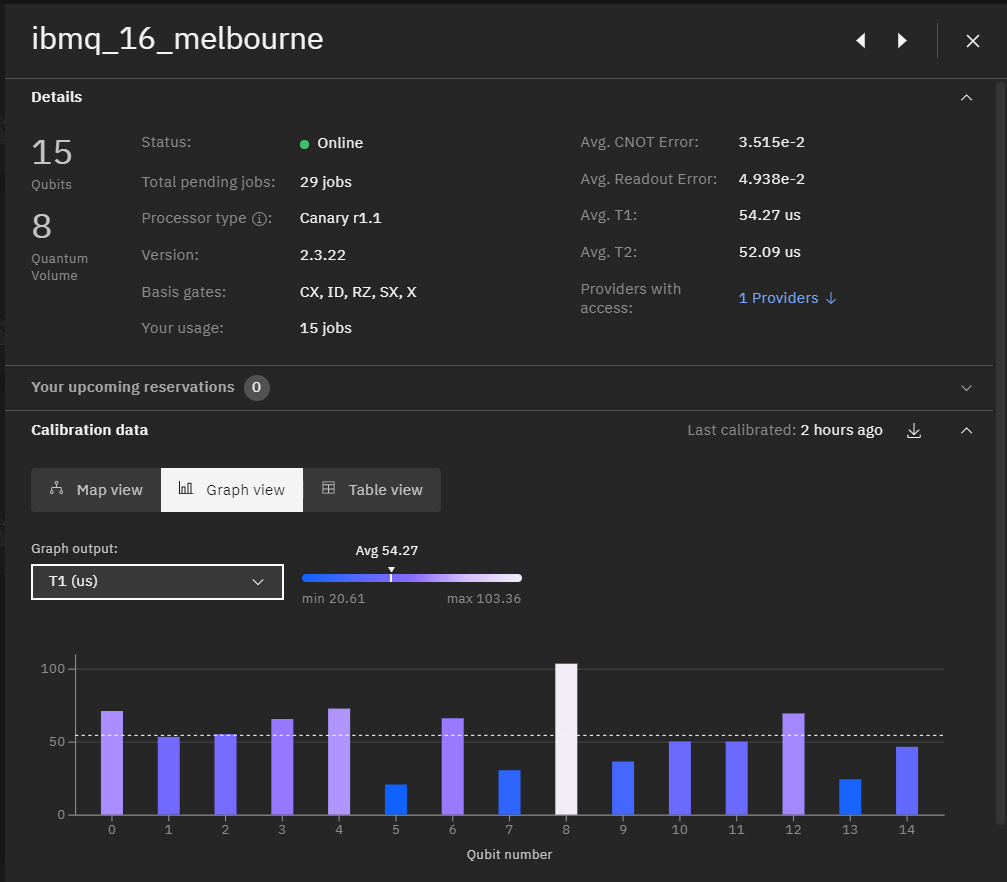The decoherence time (average T1 time) of a 15-qubits IBM Quantum computer on the Cloud “ibmq_16_melbourne”.

# Bra-ket Notation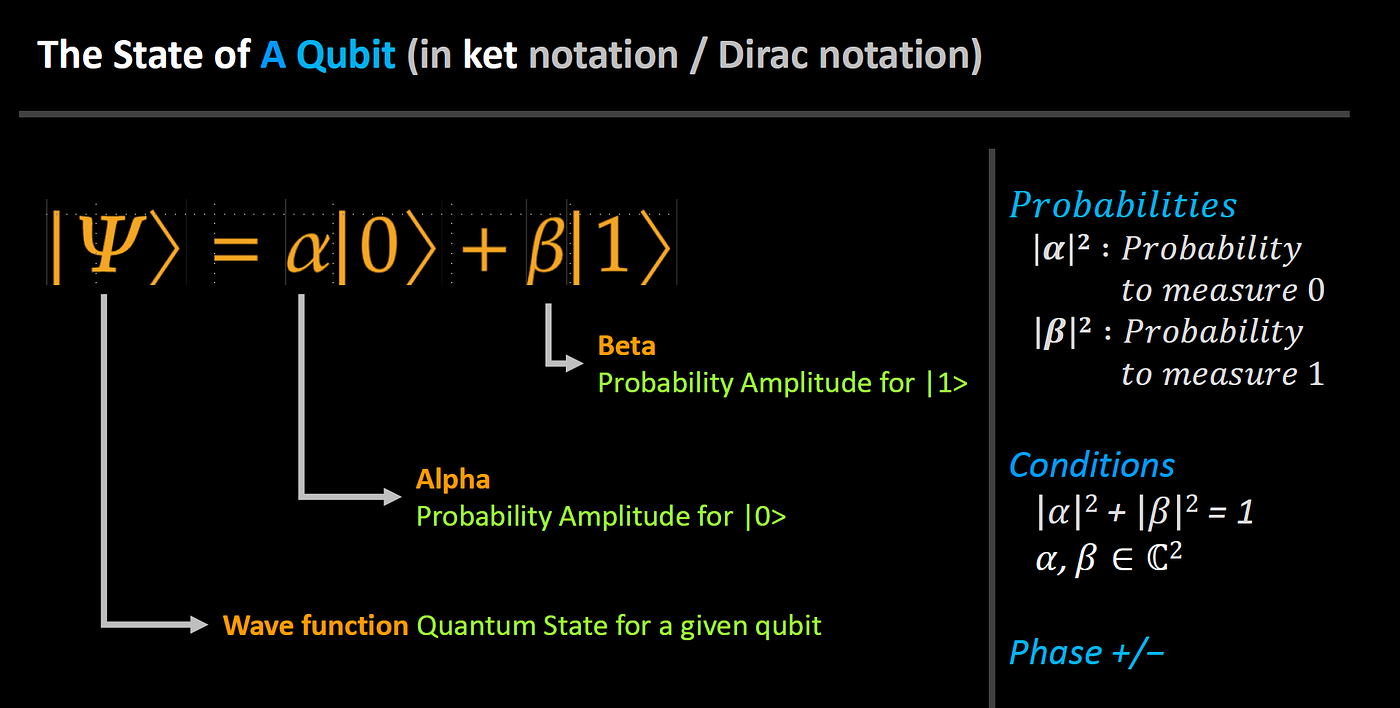A quantum state in ket notation, |Ψ>.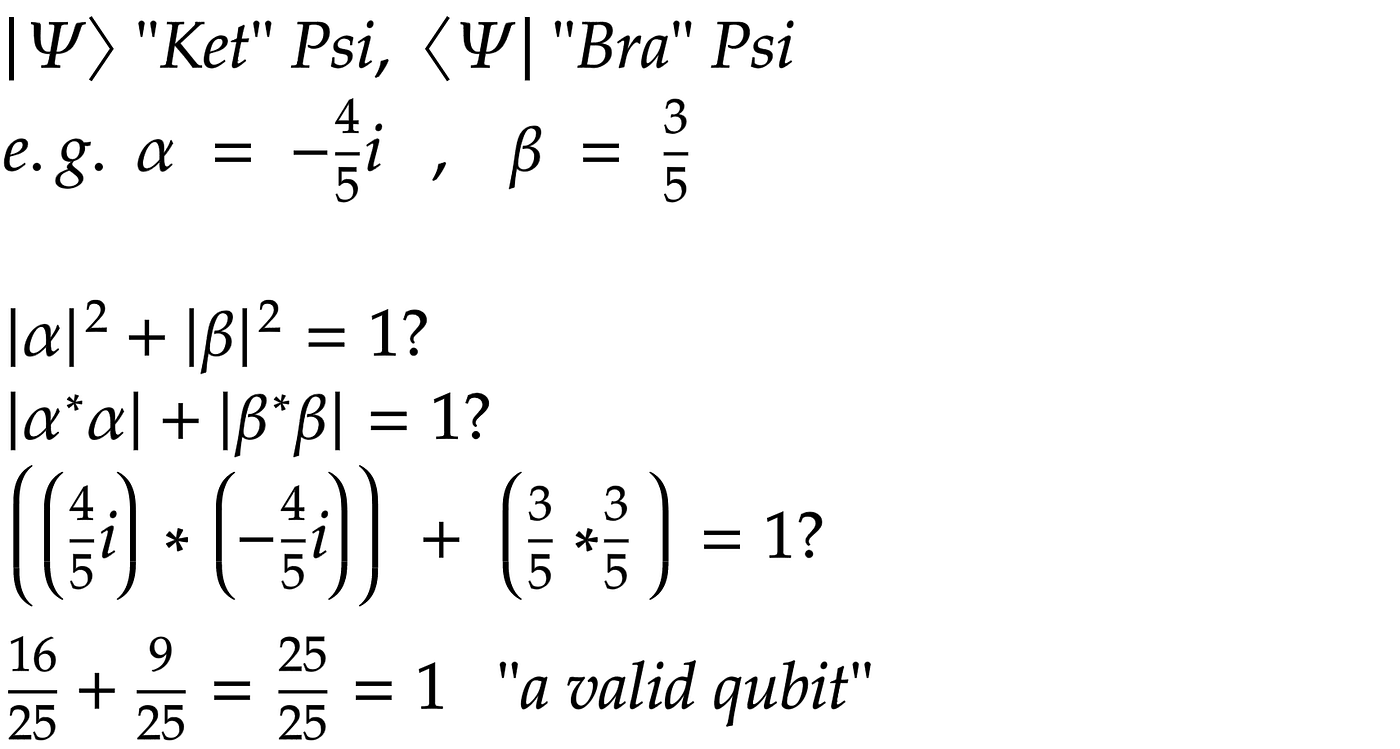Bra <Ψ| and ket |Ψ> notation, thus “Bra-ket” notation, 1 of 2.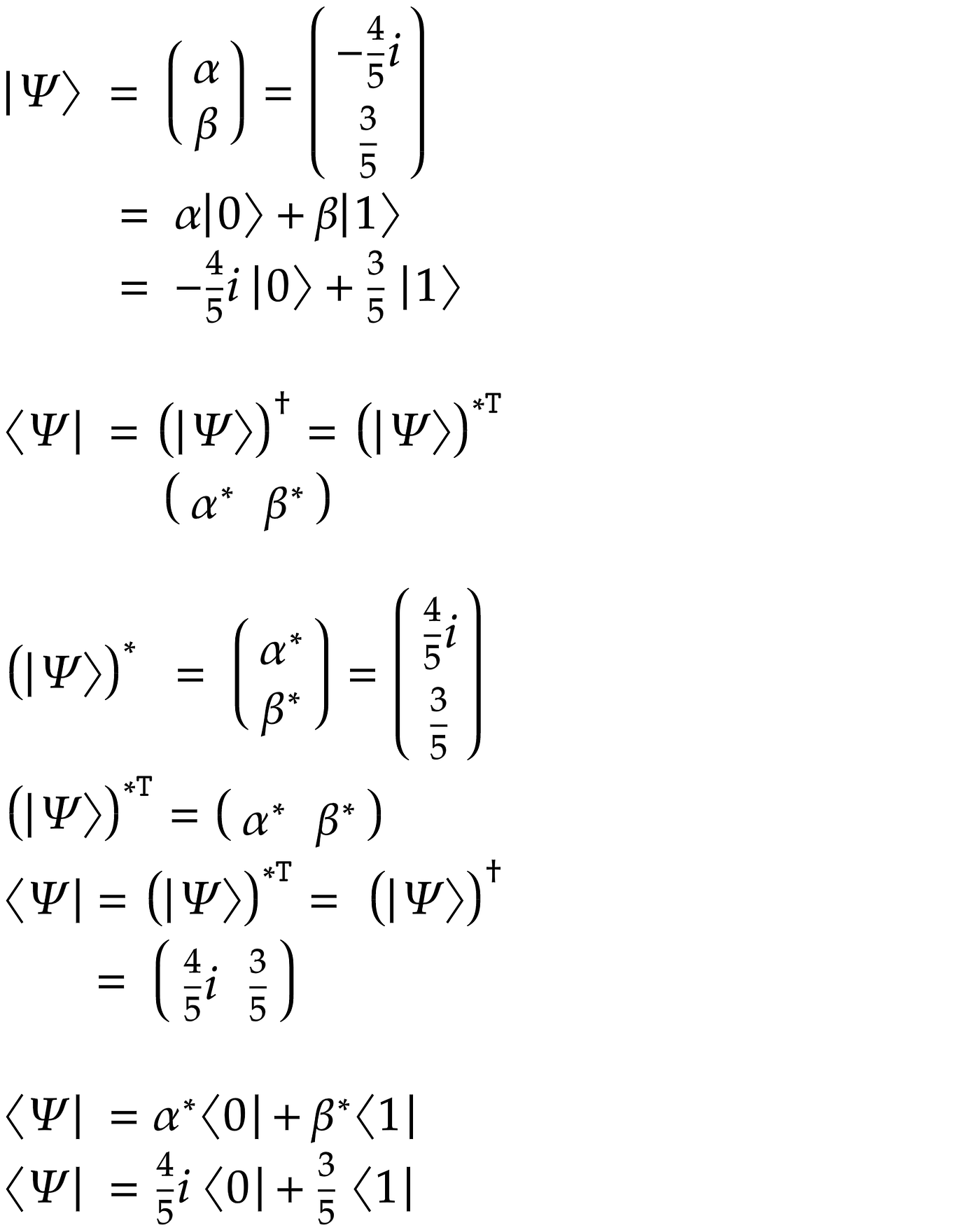Bra <Ψ| and ket |Ψ> notation, thus “Bra-ket” notation, 2 of 2.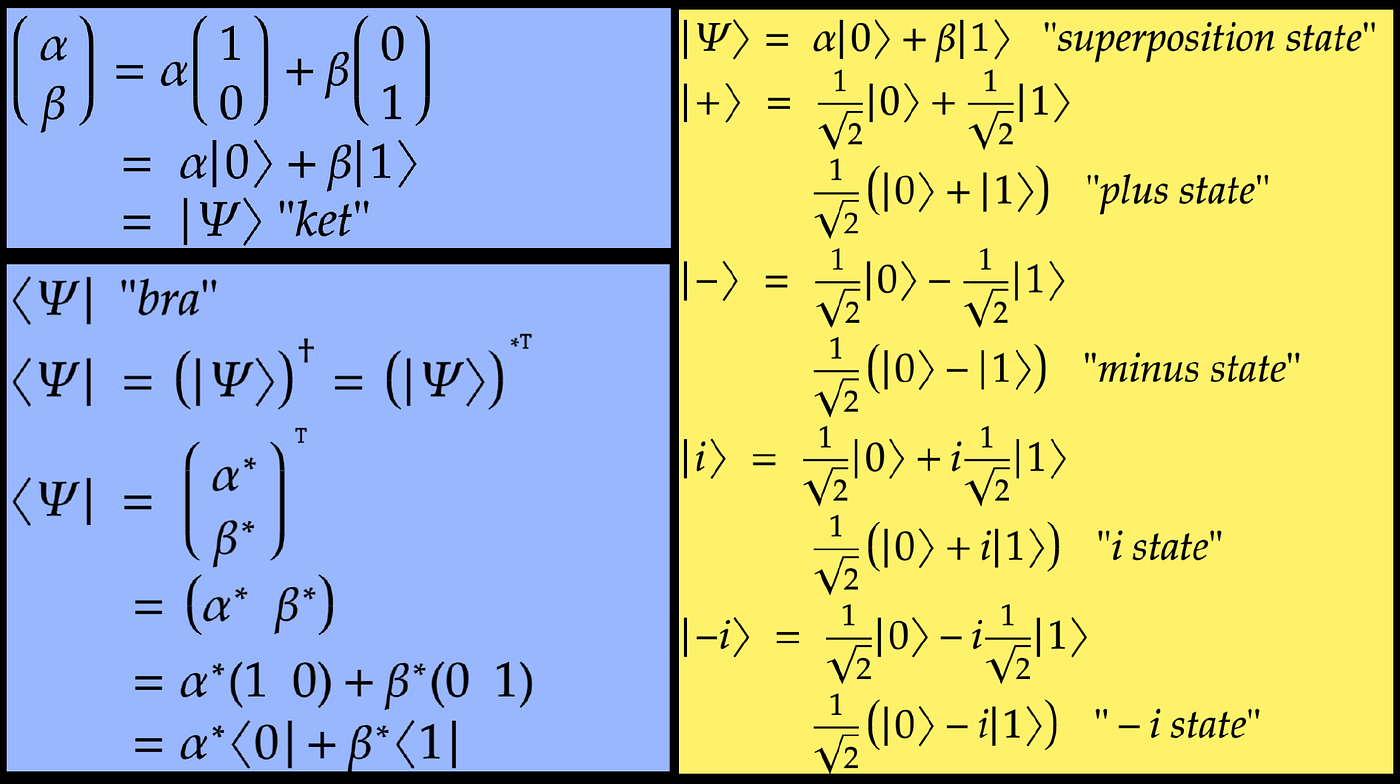Bra <Ψ| and ket |Ψ> notation, thus Bra-ket notation. Other possible quantum states are plus (|+>), minus (|->), i (|i>), and -i (|-i>).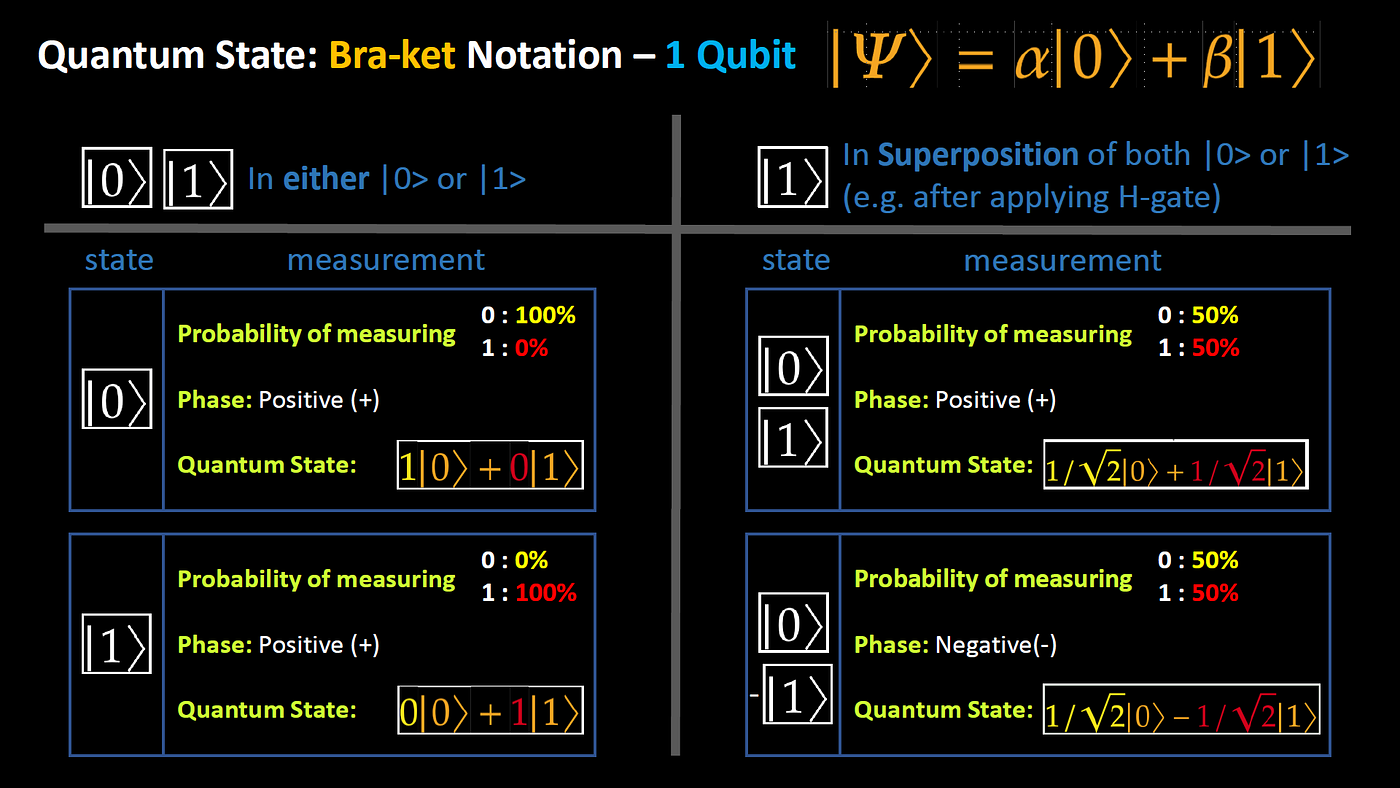When a qubit is in superposition, the probability of measuring 0 or 1 is equal at 50% and 50%, respectively.

# Bloch Sphere — Illustrating a 1-qubit state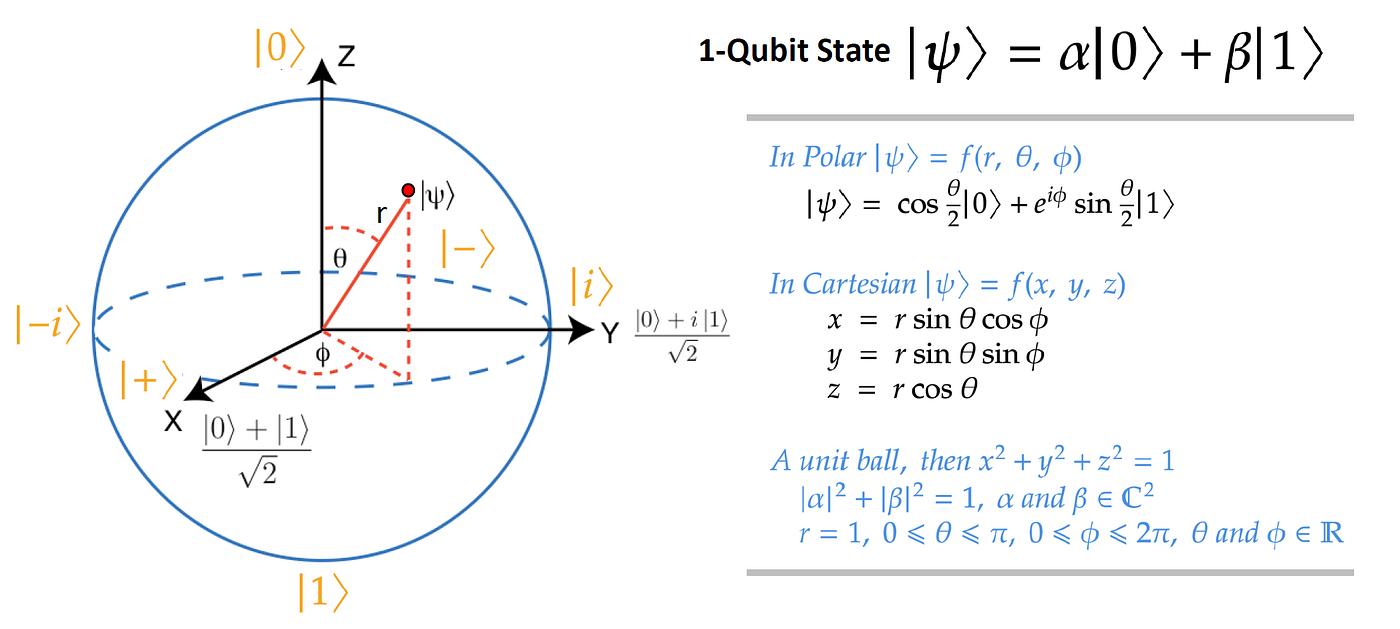The Atoms of Computation: The Bloch Sphere of a qubit (IBM, 2021b).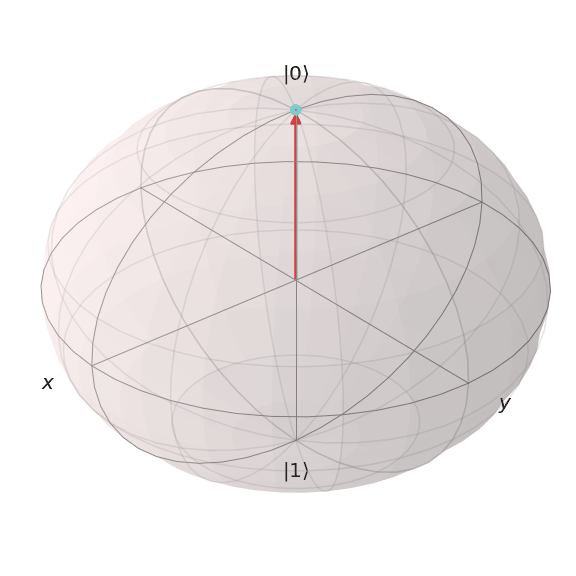Quantum state transitions for Superposition. |0> to |+> and |1> to |->.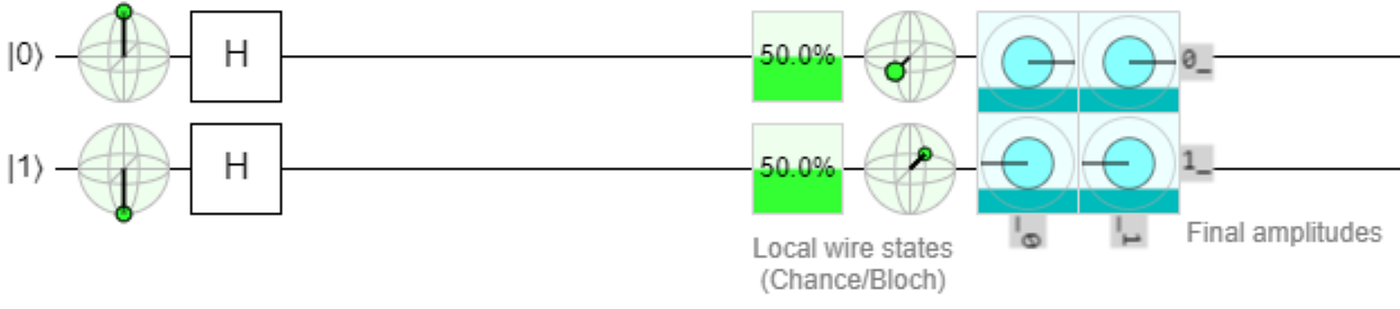An illustration of two independent qubits to illustrate Superposition: qubit 0 and qubit 1. The transition for qubit 0 is from state |0> to |+> (by applying H-gate), while qubit 1 from state |1> to |-> (also by applying H-gate). Illustrated by an online tool, Quirk (Strilanc, 2019).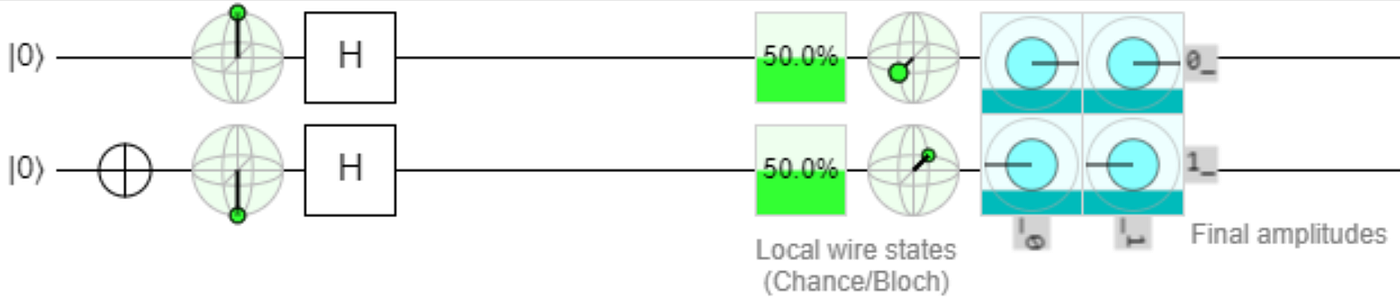An illustration of two independent qubits to illustrate Superposition: qubit 0 and qubit 1. The transition for qubit 0 is from state |0> to |+> (by applying H-gate), while qubit 1 from state |1> to |-> (also by applying H-gate). Note that, in order to bring qubit 0 to state |1>, an X-gate is applied to the |0> state). Illustrated by an online tool, Quirk (Strilanc, 2019).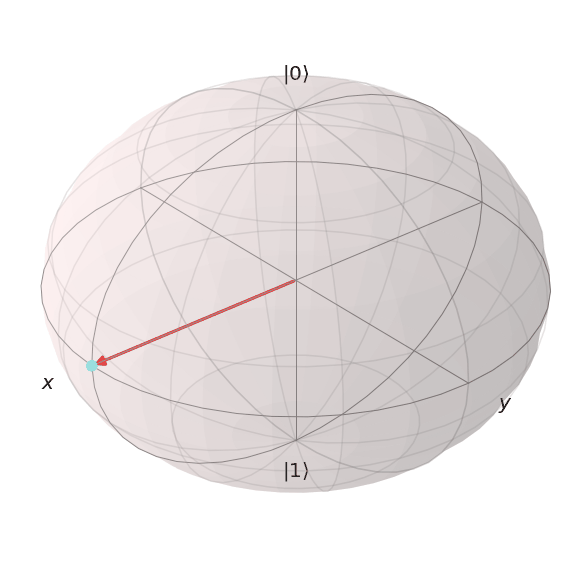Quantum state transitions from |+> to |-> and from |-> to |+>.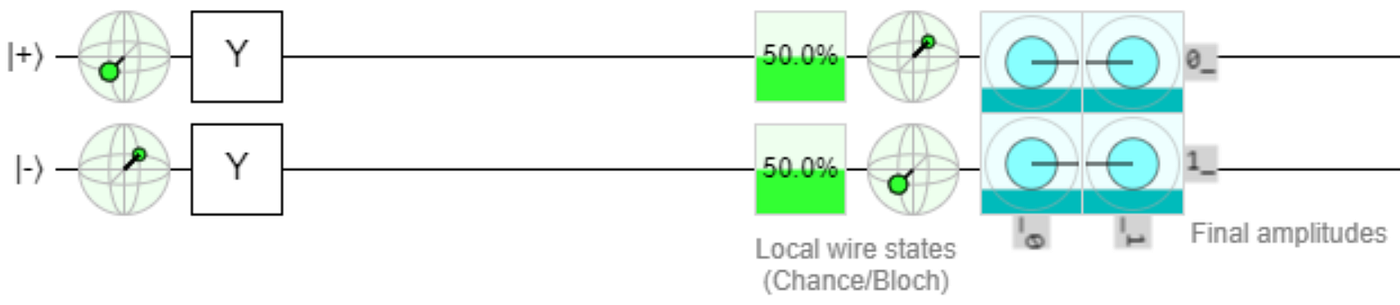An illustration of two independent qubits: qubit 0 and qubit 1. The transition for qubit 0 is from state |+> to |-> (by applying Z-gate, or Y-gate). Illustrated by an online tool, Quirk (Strilanc, 2019).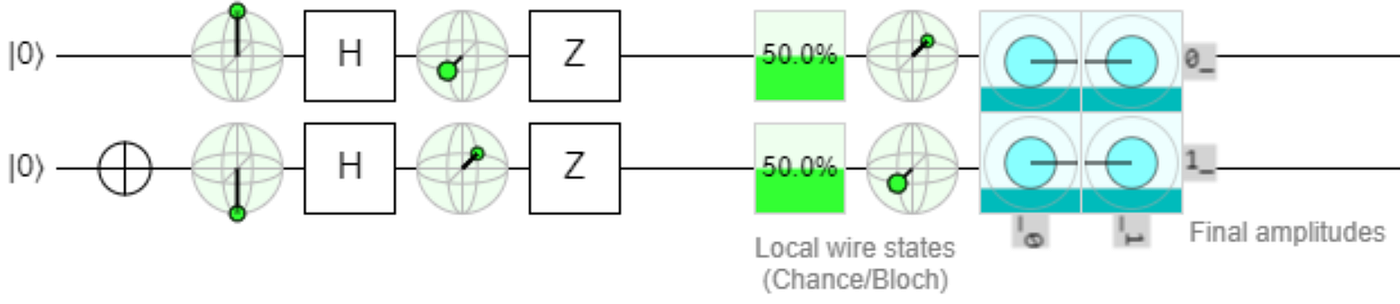An illustration of two independent qubits: qubit 0 and qubit 1. The transition for qubit 0 is from state |+> to |-> (by applying Z-gate, or Y-gate), while qubit 1 from state |-> to |+> (also by applying Z-gate, or Y-gate). Note that, in order to bring qubit 0 to state |+> and qubit 1 to |->, an H-gate is applied to both independently, by initially setting the state for qubit 0 = |0> and qubit 1 = |1>. Illustrated by an online tool, Quirk (Strilanc, 2019).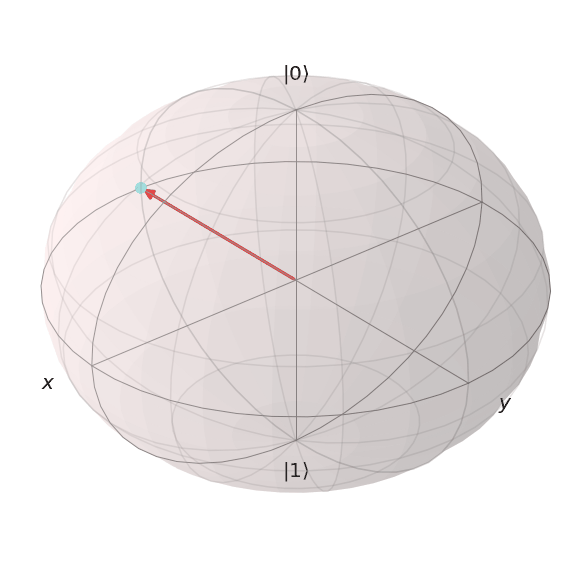Quantum state transitions from |-i> to |i>.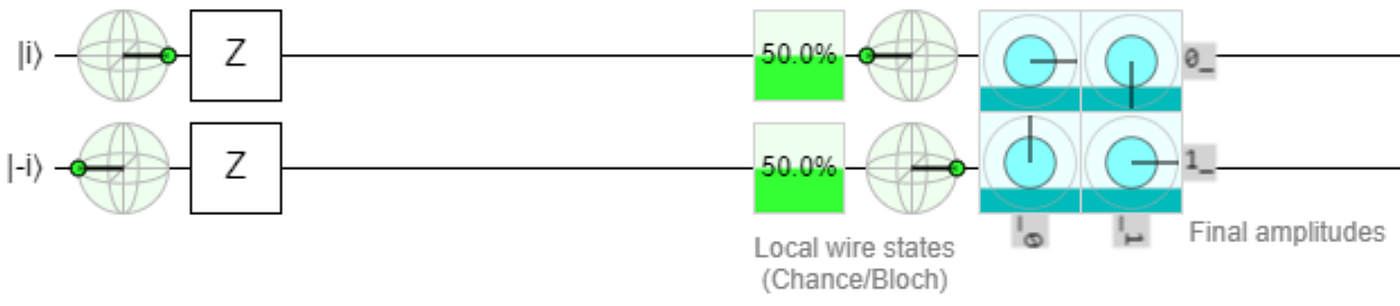An illustration of two independent qubits: qubit 0 and qubit 1. The transition for qubit 0 is from state |i> to -|i> (by applying Z-gate), while qubit 1 is from the state -|i> to |i> (also by applying Z-gate). Illustrated by an online tool, Quirk (Strilanc, 2019).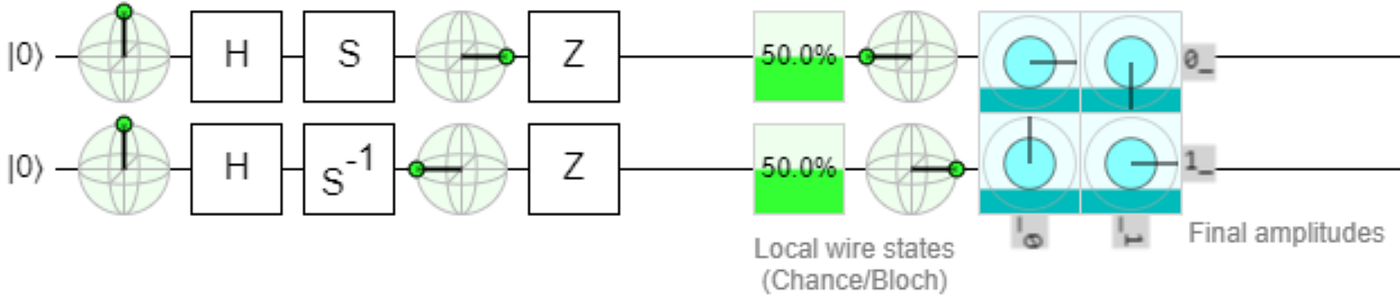An illustration of two independent qubits: qubit 0 and qubit 1. The transition for qubit 0 is from state |i> to -|i> (by applying Z-gate), while qubit 1 is from the state -|i> to |i> (also by applying Z-gate). Note that, in order to bring qubit 0 to state |+> and qubit 1 to |->, an H-gate followed by an S-gate (or S-gate inverse) are applied to both independently, by initially setting the states for both qubits = |0>. Illustrated by an online tool, Quirk (Strilanc, 2019).

# Measurement — with IBM Quantum

`Before execution, the submitted quantum circuit is translated to a series of hardware-specific supported basis gates for the specific Quantum computer. E.g., for the 27 qubits IBM Quantum computer called "ibmq_toronto" (IBM, 2021a), the supported basis gates are CX, ID, RZ, SX, and X. While for for the single qubit IBM Quantum computer called "ibmq_armonk", the supported basis gates are ID, RZ, SX, and X.`